Paul Ryan Developer hailing from Ireland. Loves all things JS and also starting to fall in love with SVGs!

# Know your JavaScript data structures

10 min read 2977Editor’s note: This article was updated in June 2021 to reflect reader-reported corrections and suggestions as well as updates to the code.

## What are JavaScript data structures?

JavaScript data structures are often overlooked — or, rather, we don’t think about them much. The problem with ignoring data structures is that for many companies, you are usually required to have a deep understanding of how to manage your data. A strong grasp of data structures will also help you in your day-to-day job as you approach problems.

In this article, the data structures we will be discussing and implementing are:

• Stack
• Queue
• Hash table
• Trees

## Stack

The first JavaScript data structure we are discussing is the stack. This is quite similar to the queue, and you may have heard of the `call stack` before, which is what JavaScript uses to handle events.

Visually, the stack looks like this: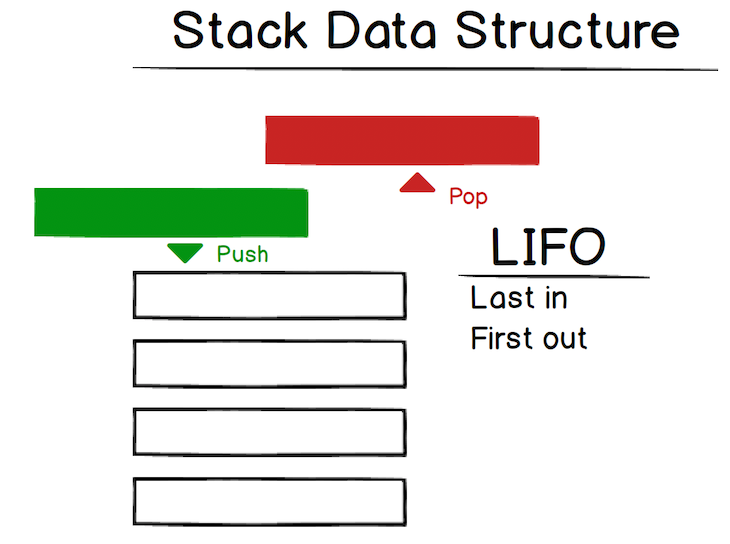So when you have a stack, the last item you pushed on the stack will be the first one removed. This is referred to as last-in, first-out (LIFO). The back button in web browsers is a good example: each page you view is added to the stack, and when you click back, the current page (the last one added) is popped from the stack.

That is enough theory. Let’s get into some code. For the stack, we are going to use an object and pretend that JavaScript doesn’t have an array data structure. Then when we move onto the queue data structure, we will use an array.

```class Stack {
constructor() {
// create our stack, which is an empty object
this.stack = {}
}
// this method will push a value onto the top of our stack
push(value) {

}
// this method is responsible for popping off the last value and returning it
pop() {

}

// this will peek at the last value added to the stack
peek() {

}
}```

I’ve added comments to the above code, so hopefully, you are with me up to this point. The first method we will implement is the `push` method.

Let’s think about what we need this method to do:

• We need to accept a value
• We then need to add that value to the top of our stack
• We also should track the length of our stack so we know our stack’s index

It would be great if you could try this yourself first, but if not, the complete `push` method implementation is below:

```class Stack {
constructor() {
this._storage = {};
this._length = 0; // this is our length
}

push(value) {
// so add the value to the top of our stack
this._storage[this._length] = value;
// since we added a value, we should also increase the length by 1
this._length++;
}
/// .....
}```

I bet it was easier than you thought — with lot of these structures, they sound more complicated than they actually are.

Now let’s get to the `pop` method. The goal with the `pop` method is to remove the last value that was added to our stack and then return that value. Try this yourself first if you can, otherwise just continue on to see the solution:

```class Stack {
constructor() {
this._storage = {};
this._length = 0;
}

pop() {
const lastValIndex = this._length - 1;
if (lastValIndex >= 0) {
// we first get the last val so we have it to return
const lastVal = this._storage[lastValIndex];
// now remove the item which is the length - 1
delete this._storage[lastValIndex];
// decrement the length
this._length--;
// now return the last value
return lastVal;
}
return false;
}
}```

Cool! Nearly there. The last thing we need to do is the `peek` function, which looks at the last item in the stack. This is the easiest function: we simply return the last value. Implementation is:

```class Stack {
constructor() {
this._storage = {};
this._length = 0;
}

peek() {
const lastValIndex = this._length - 1;
const lastVal = this._storage[lastValIndex];
return lastVal;
}
}```

This is pretty similar to the `pop` method, but this time, we do not remove the last item.

Yes! That is our first data structure covered. Now let’s move on to the queue, which is quite similar to the stack.

## Queue

The queue is the next structure we will discuss — hopefully the stack is still fresh in your brain because the queue is quite similar. The key difference between the stack and the queue is that the queue is first-in, first-out (FIFO). There have been a few comments on this article asking why not use an array here, so as a contrast to the above, we will use an array for this data structure.

Visually, we can represent it like this: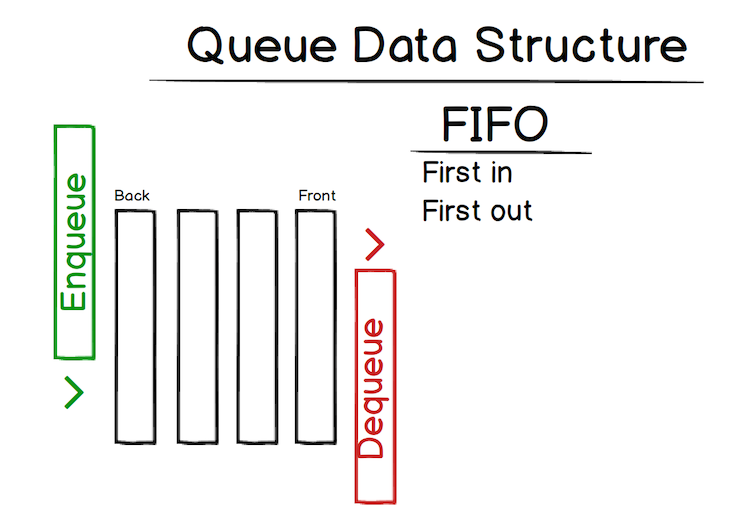The two big actions are `enqueue` and `dequeue`. We add to the back and remove from the front. Let’s get into implementing a queue to get a better understanding. I had previously used an object here, but I have updated it now to use an array. For the stack data structure, you can also do this approach.

### More great articles from LogRocket:

The core structure of our code will look like this:

```class Queue {
constructor() {
// array to hold our values
this.queue = [];
// length of the array - could also track this with queue.length
this.length = 0;
}

enqueue(value) {

}

dequeue() {

}

peek() {

}
}```

Let’s first implement our `enqueue` method. Its purpose is to add an item to the back of our queue.

```enqueue(value) {
// add a value to the back of the queue
this.queue.push(value);
// update our length (can also be tracked with queue.length)
this.length++;
}```

This is quite a simple method that adds a value to the end of our queue, but you may be a little confused by `this.queue[this.length + this.head] = value;`.

Let’s say our queue looked like this: `{14 : 'randomVal'}`. When adding to this, we want our next key to be `15`, so it would be length(1) + head(14), which gives us `15`.

The next method to implement is the `dequeue` method (remove an item from the front of our queue):

```dequeue() {
// if we have any values
if (this.length > 0) {
// remove an element from the front of the queue
this.queue.shift();
// decrement the length
this.length--;
}
}```

The final method to implement is the `peek` method, which is an easy one (return the first value of the queue):

```peek() {
if(this.length > 0) {
return this.queue;
}
return null;
}```

That’s it for the queue — let’s move on to the linked list data structure.

Let’s discuss the formidable linked list. This is more complicated than our structures above, but together, we can figure it out.

The first question you might ask is why we would use a linked list. A linked list is mostly used for languages that do not have dynamic sizing arrays. Linked lists organize items sequentially, with each item pointing to the next item.

Each node in a linked list has a `data` value and a `next` value. Below, `5` is the data value, and the `next` value points to the next node, i.e., the node that has the value `10`.

Visually, the linked list data structure looks like this: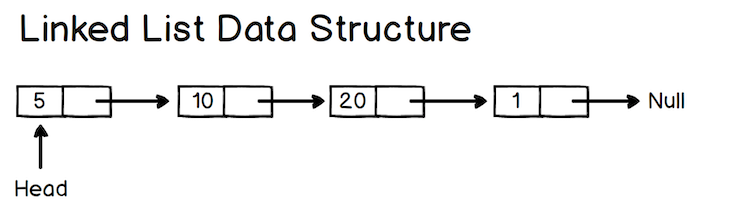As a side note, a previous pointer is called a doubly linked list.

In an object, the above `LinkedList` would look like the following: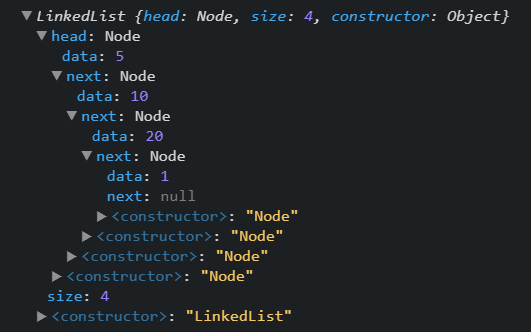You can see that the last value `1` has a `next` value of `null`, as this is the end of our `LinkedList`.

So now, how would we implement this?

The first thing we are going to create is a `Node` class.

```class Node {
constructor(data, next = null) {
this.data = data;
this.next = next;
}
}```

The above represents each node in our list.

With a class for our `Node`, the next class we need is our `LinkedList`.

```class LinkedList {
constructor() {
this.size 0;
}
}```

As explained above, our `LinkedList` has a `head`, which is first set to `null` (you could add an `arg` to your constructor to set this if you wanted). We also track the `size` of our linked list.

The first method we are going to implement is `insert`; this will add a `node` to our linked list

```// insert will add to the end of our linked list
insert(data) {
// create a node object using the data passed in
let node = new Node(data);
let current;
// if we don't have a head, we make one
} else {
// if there is already a head, then we add a node to our list
// loop until the end of our linked list (the node with no next value)
while (current.next) {
current = current.next;
}
// set the next value to be the current node
current.next = node;
}
// increment the size
this.size++;
}```

I have commented in the code above to make it easier to understand, but all we are doing is adding a `node` to the end of the linked list. We can find the end of our linked list by finding the `node` that has a `next` value of `null`.

The next method we are going to implement is `removeAt`. This method will remove a `node` at an index.

```// Remove at index
removeAt(index) {
// check if index is a positive number and index isn't too large
if (index < 0 || index > this.size) {
return;
}
// start at our head
let current = this.head;
// keep a reference to the previous node
let previous;
// count variable
let count = 0;
// if index is 0, then point the head to the item second (index 1) in the list
if (index === 0) {
} else {
// loop over the list and
while (count < index) {
// first increment the count
count++;
// set previous to our current node
previous = current;
// now set our current node to the next node
current = current.next;
}
// update the next pointer of our previous node to be the next node
previous.next = current.next;
}
// since we removed a node we decrement, the size by 1
this.size--;
}```

So the method above will remove a node at a specific index. It does this by updating the next value to point at the next node in the list until we reach the index. This means that no node will be pointing at the node at the index, so it will be removed from our list.

The final (easiest) method left to do is `clearList`.

```clearList() {
this.size = 0;
}```

This just resets everything back to the start. There are lots of methods you can add to your linked list, but the above sets down the core fundamentals that you need to know.

## Hash table

So the second-to-last data structure we are tackling is the mighty hash table. I purposefully placed this after the `LinkedList` explanation, as they are not a million miles away from each other.

A hash table is a data structure that implements an associative array, which means it maps keys to values. A JavaScript object is a hash table, as it stores key-value pairs.

Visually, this can be represented like so: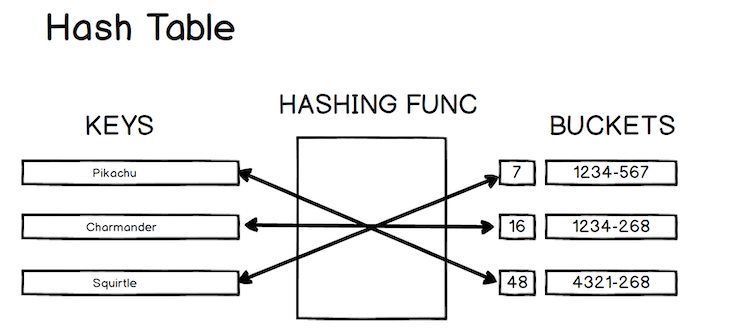Before we start talking about how to implement the hash table, we need to discuss the importance of the hashing function. The core concept of the hashing function is that it takes an input of any size and returns a hash code identifier of a fixed size.

`hashThis('i want to hash this') => 7`

The hashing function can be very complicated or straightforward. Each of your files on GitHub are hashed, which makes the lookup for each file quite fast. The core idea behind a hashing function is that given the same input will return the same output.

With the hashing function covered, it’s time to talk about how we would implement a hash table.
The three operations we will discuss are `insert`, `get`, and, finally, `remove`.

The core code to implement a hash table is as follows:

```class HashTable {
constructor(size) {
// define the size of our hash table, which will be used in our hashing function
this.size = size;
this.storage = [];
}
insert(key, value) { }
get() {}
remove() {}
// this is how we will hash our keys
myHashingFunction(str, n) {
let sum = 0;
for (let i = 0; i < str.length; i++) {
sum += str.charCodeAt(i) * 3;
}
return sum % n;
}
}```

Now let’s tackle our first method, which is `insert`. The code to `insert` into a hash table is as follows (to keep things simple, this method will handle collisions but not duplicates):

```insert(key, value) {
// will give us an index in the array
const index = this.myHashingFunction(key, this.size);
// handle collision - hash function returns the same
// index for a different key - in complicated hash functions it is very unlikely
// that a collision would occur
if (!this.storage[index]) {
this.storage[index] = [];
}
// push our new key value pair
this.storage[index].push([key, value]);
}
```

So if we were to call the insert method like so:

```const myHT = new HashTable(5);
myHT.insert("a", 1);
myHT.insert("b", 2);```

What do you think our hash table would look like?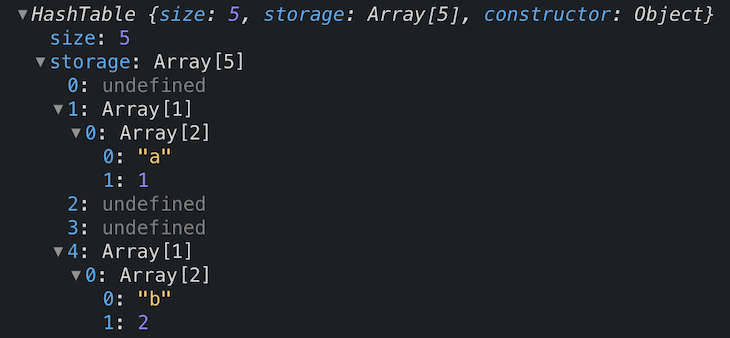You can see our key-value pair has been inserted into our table at index `1` and `4`.

Now how would we remove a value from a hash table?

```remove(key) {
// first we get the index of our key
// remember, the hashing function will always return the same index for the same
// key
const index = this.myHashingFunction(key, this.size);
// remember we could have more than one array at an index (unlikely)
let arrayAtIndex = this.storage[index];
if (arrayAtIndex) {
// let's loop over all the arrays at that index
for (let i = 0; i < arrayAtIndex.length; i++) {
// get the pair (a, 1)
let pair = arrayAtIndex[i];
// check if the key matches the key param
if (pair === key) {
// delete the array at index
delete arrayAtIndex[i];
// job done, so break out of the loop
break;
}
}
}
}```

Regarding the above, you may be thinking, “Is this not linear time? I thought hash tables are meant to be constant?” You would be correct in thinking that, but since this situation is quite rare with complicated hashing functions, we still consider hash tables to be constant.

The final method we will implement is the `get` method. This is the same as the `remove` method, but this time, we return the `pair` rather than delete it.

``` get(key) {
const index = this.myHashingFunction(key, this.size);
let arrayAtIndex = this.storage[index];
if (arrayAtIndex) {
for (let i = 0; i < arrayAtIndex.length; i++) {
const pair = arrayAtIndex[i];
if (pair === key) {
// return the value
return pair;
}
}
}
}```

I don’t think there is a need to go through this, as it acts the same as the `remove` method.

This is a great introduction to the hash table, and as you can tell, it is not as complicated as it initially seems. This is a data structure that is used all over the place, so it is a great one to understand!

## Binary search tree

Sadly (or maybe thankfully), this is the last data structure that we will tackle — the notorious binary search tree.

When we think of a binary search tree, the three things we should think of are:

• Root: This is the very top node of a tree structure and does not have a parent
• Parent: It is a child of a node but also the parent of a node
• Child: This node is the child of a node and does not necessarily have a child

In a binary search tree, each node either has zero, one, or two children. The child on the left is called the left child, and the child on the right is the right child. In a binary search tree, the child on the left must be smaller than the child on the right.

Visually, you can picture a binary search tree like so: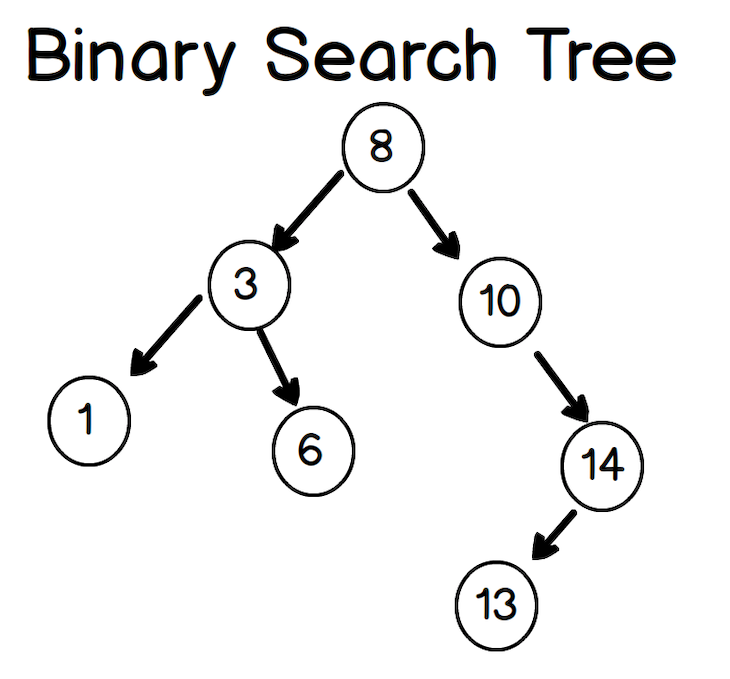The core class for a tree would look like this:

```class Tree {
constructor(value) {
this.root = null
}

// we'll implement this below
}

}```

We’ll also create a `Node` class to represent each of our nodes.

```class Node {
constructor(value, left = null, right = null) {
this.value = value;
this.left = left;
this.right = right;
}
}```

OK, let’s implement the `add` method. I have commented in the code, but if you find it confusing, just remember that all we are doing is going from our root and checking the `left` and `right` of each node.

```add(value) {
Let newNode = new Node(value);
// if we do not have a root, then we create one
if (this.root === null) {
this.root = newNode;
return this;
}
let current = this.root;
// while we have a node
while (current) {
if(value === current.value) return undefined;
// go left if our current value is greater
// than the value passed in
if (current.value > value) {
// if there is a left child, then run the
// loop again
if (current.left) {
current = current.left;
} else {
current.left = newNode;
return this;
}
}
// the value is smaller, so we go right
else {
// go right
// if there is a left child, then run the
// loop again
if (current.right) {
current = current.right;
} else {
current.right = newNode;
return this;
}
}
}
}
```

Let’s test our new `add` method like so:

```const t = new Tree();

Our tree now looks like the following: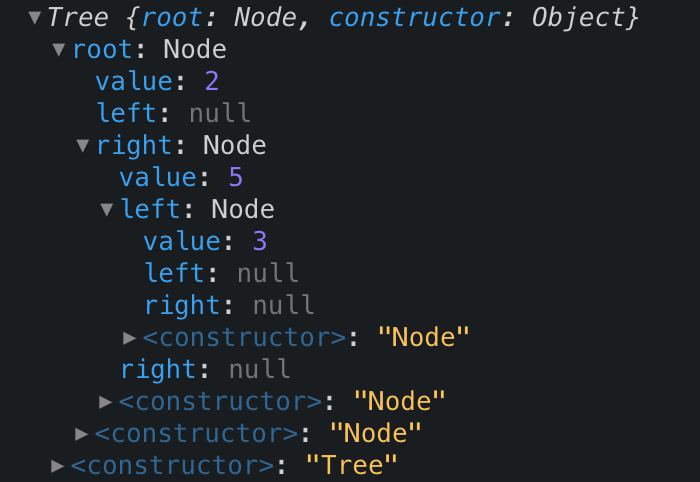So to get an even better understanding, let’s implement a method that checks if our tree contains a value.

```contains(value) {
// get the root
let current = this.root;
// while we have a node
while (current) {
// check if our current node has the value
if (value === current.value) {
return true; // leave the function
}
// we decide on the next current node by comparing our value
// against current.value - if its less go left else right
current = value < current.value ? current.left : current.right;
}
return false;
}```

`Add` and `Contains` are the two core methods of the binary search tree. An understanding of both these methods give you better perspective on how you would tackle problems at your day-to-day job.

## Conclusion

Wow, this was a long one. We have covered a lot of material in this article, and it will greatly assist you in technical interviews. I really hope you learned something (I know I have) and that you will feel more comfortable approaching technical interviews (especially the nasty white-boarding ones).

## LogRocket: Debug JavaScript errors more easily by understanding the context

Debugging code is always a tedious task. But the more you understand your errors the easier it is to fix them.

LogRocket allows you to understand these errors in new and unique ways. Our frontend monitoring solution tracks user engagement with your JavaScript frontends to give you the ability to find out exactly what the user did that led to an error.

LogRocket records console logs, page load times, stacktraces, slow network requests/responses with headers + bodies, browser metadata, and custom logs. Understanding the impact of your JavaScript code will never be easier!

.Paul Ryan Developer hailing from Ireland. Loves all things JS and also starting to fall in love with SVGs!

## 7 Replies to “Know your JavaScript data structures”

1.Nikita Bazalev says:

Stack implementation has few errors.
For example try this code:
var stack = new Stack();
stack.push(1);
stack.peek(); // –> 1
stack.peek(); // –> undefined, because this._length became -1
Same problem with pop() method – you decrement this._length three times

2.Dave says:

`–this.length` is used in error 3 times – decrementing the values instead of retrieving the position. It’s a pretty fundamental error for a data structures tutorial

3.Paul Ryan says:

Yes I will be making an update. Errors can happen when writing such a long form article .

4.Milos says:

Hi, in Linked list when I want to remove last node(which is tail), the value of tail stays the same even if it’s deleted. Would this be good way to chage value of the tail? Im still learning.

if(currentNode === this.tail){
this.tail = previousNode;
previousNode.next = currentNode.next;
return;
}

5.Dave says:

There’s a few bugs in the Queue implementation e.g. the `dequeue()` method doesn’t have a `return` statement, so the `firstVal` isn’t returned. If `enqueue(val)` is called multiple times the length can become a negative number, meaning subsequent `peek()` calls return undefined, even after adding values

6.Evgeniia says:

Why do not use simply push() and pop() for a FIFO Queue? Or Queue must be an array?

7.Paul Ryan says:

I updated the article to use an array for the queue, as a note though you wouldn’t use `push` you would use `unshift`